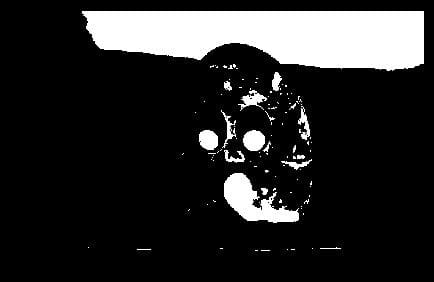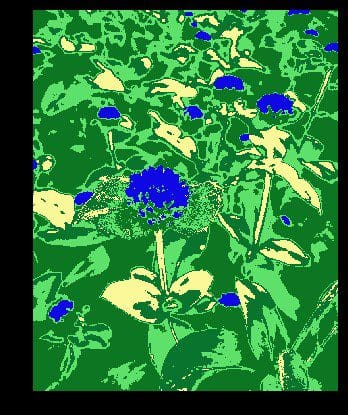# Basic Image Data Analysis Using Python – Part 4

Accessing the internal component of digital images using Python packages helps the user understand its properties, as well as its nature.
By Mohammed Innat, Khulna University of Engineering & Technology.__data analysis Python fig 1" width="100%" srcset="https://www.kdnuggets.com/wp-content/uploads/image-data-analysis-python-fig1.jpg 700w, https://www.kdnuggets.com/wp-content/uploads/image-data-analysis-python-fig1-300x135.jpg 300w" sizes="(max-) 100vw, 700px" />

Previously, we’ve seen some of the very basic image analysis operations in Python. In this last part of basic image analysis, we’ll go through some of the following contents.

Following contents is the reflection of my completed academic image processing course in the previous term. So, I am not planning on putting anything into production sphere. Instead, the aim of this article is to try and realize the fundamentals of a few basic image processing techniques. For this reason, I am going to stick to using SciKit-Image - numpy mainly to perform most of the manipulations, although I will use other libraries now and then rather than using most wanted tools like OpenCV :

I wanted to complete this series into two section but due to fascinating contents and its various outcome, I have to split it into four parts. You can find the first three here:

Part 1 | Part 2 | Part 3

Now, let's get started!

### Thresholding

Ostu’s Method

Thresholding is a very basic operation in image processing. Converting a greyscale image to monochrome is a common image processing task. And, a good algorithm always begins with a good basis!

Otsu thresholding is a simple yet effective global automatic thresholding method for binarizing grayscale images such as foregrounds and backgrounds. In image processing, Otsu’s thresholding method (1979) is used for automatic binarization level decision, based on the shape of the histogram. It is based entirely on computation performed on the histogram of an image.

The algorithm assumes that the image is composed of two basic classes: Foreground and Background. It then computes an optimal threshold value that minimizes the weighted within class variances of these two classes.

Otsu threshold is used in many applications from medical imaging to low-level computer vision. It’s many advantages and assumptions.

Mathematical Formulation of Otsu method. This will redirect you to my homepage where we explained mathematics behind Otsu method.

Algorithm

If we incorporate a little math into that simple step-wise algorithm, such an explanation evolves:

• Compute histogram and probabilities of each intensity level.
• Set up initial wiand μi.
• Step through from threshold t = 0 to t = L-1:
• update: wi and μi
• compute: σ2b(t)

The Desired threshold corresponds to the maximum value of σ2b(t).

```
import numpy as np
import imageio
import matplotlib.pyplot as plt

plt.figure(figsize=(7,7))
plt.axis('off')
plt.imshow(pic);
```__data analysis Python fig 2" width="50%" srcset="https://www.kdnuggets.com/wp-content/uploads/image-data-analysis-python-fig2.jpg 434w, https://www.kdnuggets.com/wp-content/uploads/image-data-analysis-python-fig2-300x195.jpg 300w" sizes="(max-) 100vw, 434px" />

```
def otsu_threshold(im):

# Compute histogram and probabilities of each intensity level
pixel_counts = [np.sum(im == i) for i in range(256)]

# Initialization
s_max = (,)

for threshold in range(256):

# update
w_0 = sum(pixel_counts[:threshold])
w_1 = sum(pixel_counts[threshold:])

mu_0 = sum([i * pixel_counts[i] for i in range(,threshold)]) / w_0 if w_0 > 0 else
mu_1 = sum([i * pixel_counts[i] for i in range(threshold, 256)]) / w_1 if w_1 >  else

# calculate - inter class variance
s = w_0 * w_1 * (mu_0 - mu_1) ** 2

if s > s_max:
s_max = (threshold, s)

return s_max[]
def threshold(pic, threshold):
return ((pic > threshold) * 255).astype('uint8')

gray = lambda rgb : np.dot(rgb[... , :3] , [0.21 , 0.72, 0.07])

plt.figure(figsize=(7,7))
plt.imshow(threshold(gray(pic), otsu_threshold(pic)), cmap='Greys')
plt.axis('off');
```Nice but not Great. Otsu’s method exhibits the relatively good performance if the histogram can be assumed to have bimodal distribution and assumed to possess a deep and sharp valley between two peaks.

So, now if the object area is small compared with the background area, the histogram no longer exhibits bimodality and if the variances of the object and the background intensities are large compared to the mean difference, or the image is severely corrupted by additive noise, the sharp valley of the gray level histogram is degraded.

As a result, the possibly incorrect threshold determined by Otsu’s method results in the segmentation error. But we can further improve Otsu’s method.

### KMeans Clustering

k-means clustering is a method of vector quantization, originally from signal processing, that is popular for cluster analysis in data mining.

In Otsu thresholding, we found the threshold which minimized the intra-segment pixel variance. So, rather than looking for a threshold from a gray level image, we can look for clusters in color space, and by doing so we end up with the K-means clustering technique.

```
from sklearn import cluster

import matplotlib.pyplot as plt

plt.figure(figsize=(7,7))
plt.imshow(pic)
plt.axis('off');
```For clustering the image, we need to convert it into a two-dimensional array.

```
x, y, z = pic.shape
pic_2d = pic.reshape(x*y, z)```

Next, we use scikit-learn’s cluster method to create clusters. We pass n_clusters as 5 to form five clusters. The clusters appear in the resulting image, dividing it into five parts with distinct colors.

The clustering number 5 was chosen heuristically for this demonstration. One can change the number of clusters to visually validate image with different colors and decide that closely matches the required number of clusters.

```
%%time

# fit on the image with cluster five
kmeans_cluster = cluster.KMeans(n_clusters=5)
kmeans_cluster.fit(pic_2d)

cluster_centers = kmeans_cluster.cluster_centers_
cluster_labels = kmeans_cluster.labels_
Wall time: 16.2 s```

Once the clusters are formed, we can recreate the image with the cluster centers and labels to display the image with grouped patterns.

```
plt.figure(figsize=(7,7))
plt.imshow(cluster_centers[cluster_labels].reshape(x, y, z))
plt.axis('off');
```### Line Detection

Hough Transform
Hough Transform is a popular technique to detect any shape if we can represent that shape in mathematical form. It can detect the shape even if it is broken or distorted a little bit. We won’t go too deeper to analyze the mechanism of Hough transform rather than giving intuitive mathematical description before implementing it on code and also provide some resource to understand it more in details.

Mathematical Formulation of Hough Transform. This will redirect you to my homepage where we explained mathematics behind Hough Transform method.```
where
ρ = distance from origin to the line. [-Dmax, Dmax]
Dmax is the diagonal length of the image.

θ = angle from origin to the line. [-90° to 90°]
```

Algorithm

• Corner or edge detection
• ρrange and θ range creation
• ρ : -Dmax to Dmax
• θ : -90 to 90
• Hough accumulator
• 2D array with the number of rows equal to the number of ρvalues and the number of columns equal to the number of θ
• Voting in the accumulator
• For each edge point and for each θ value, find the nearest ρvalue and increment that index in the accumulator.
• Peak finding
• Local maxima in the accumulator indicate the parameters of the most prominent lines in the input image.
```
def hough_line(img):
# Rho and Theta ranges
thetas = np.deg2rad(np.arange(-90.0, 90.0))
width, height = img.shape
diag_len = int(np.ceil(np.sqrt(width * width + height * height)))   # Dmax
rhos = np.linspace(-diag_len, diag_len, diag_len * 2.0)

# Cache some resuable values
cos_t = np.cos(thetas)
sin_t = np.sin(thetas)
num_thetas = len(thetas)

# Hough accumulator array of theta vs rho
accumulator = np.zeros((2 * diag_len, num_thetas), dtype=np.uint64)
y_idxs, x_idxs = np.nonzero(img)  # (row, col) indexes to edges

# Vote in the hough accumulator
for i in range(len(x_idxs)):
x = x_idxs[i]
y = y_idxs[i]

for t_idx in range(num_thetas):
# Calculate rho. diag_len is added for a positive index
rho = round(x * cos_t[t_idx] + y * sin_t[t_idx]) + diag_len
accumulator[rho, t_idx] += 1
return accumulator, thetas, rhos
```

### Edge Detection

Edge detection is an image processing technique for finding the boundaries of objects within images. It works by detecting discontinuities in brightness. Common edge detection algorithms include

• Sobel
• Canny
• Prewitt
• Roberts and
• fuzzy logic methods.

Here, We’ll cover one of the most popular methods, which is the Canny Edge Detection.

Canny Edge Detection

A multi-stage edge detection operation capable of detecting a wide range of edges in images. Now, the Process of Canny edge detection algorithm can be broken down into 5 different steps:

1. Apply Gaussian Filter
2. Find the intensity gradients
3. Apply non-maximum suppression
4. Apply double threshold
5. Track edge by hysteresis.